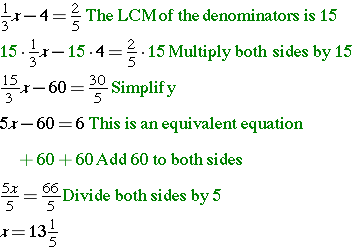# Two-Step Equations with Fractions and Decimals

CHECK THIS OUT! Did you know that you can rewrite decimal equations and fraction equations as whole number equations simply by using the Distributive Property? This strategy makes solving these problems so much simpler! Look at the following examples to see if this is a strategy that you might want to use when solving two-step fraction or decimal problems.

### Example 1: Writing Equivalent Equations without Fractions

Write an equivalent equation forthat does not contain fractions. Then solve that equation.### Example 2: Writing Equivalent Equations without Decimals

1.5x + 0.75 = 14.25

• 1.5x + 0.75 = 14.25  The equation has decimals to the hundredths, so multiply both sides by 100.
• 100(1.5x + 0.75) = 100(14.25)  Use the Distributive Property.
• 150x + 75 = 1425  This is an equivalent equation without decimals.
– 75      – 75   Subtract 75 from both sides
• 150x/150 = 1350/150  Divide both sides by 150.
• x = 9

You can use number properties to help solve any two-step equation with rational numbers!

(source)